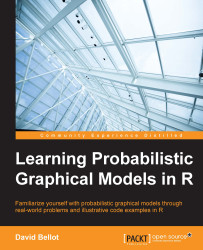•#### Learning Probabilistic Graphical Models in R#### Overview of this book

Probabilistic graphical models (PGM, also known as graphical models) are a marriage between probability theory and graph theory. Generally, PGMs use a graph-based representation. Two branches of graphical representations of distributions are commonly used, namely Bayesian networks and Markov networks. R has many packages to implement graphical models. We’ll start by showing you how to transform a classical statistical model into a modern PGM and then look at how to do exact inference in graphical models. Proceeding, we’ll introduce you to many modern R packages that will help you to perform inference on the models. We will then run a Bayesian linear regression and you’ll see the advantage of going probabilistic when you want to do prediction. Next, you’ll master using R packages and implementing its techniques. Finally, you’ll be presented with machine learning applications that have a direct impact in many fields. Here, we’ll cover clustering and the discovery of hidden information in big data, as well as two important methods, PCA and ICA, to reduce the size of big problems.
Learning Probabilistic Graphical Models in RCreditswww.PacktPub.comPrefaceFree Chapter
Probabilistic ReasoningExact InferenceLearning ParametersBayesian Modeling – Basic ModelsApproximate InferenceBayesian Modeling – Linear ModelsProbabilistic Mixture ModelsAppendixIndex## Markov Chain Monte-Carlo

MCMC methods have their origin in physics with the work of Metropolis, Ulam, and Rosenbluth. It was in the 1980s that they began to have a significant impact on statistics. Many MCMC algorithms and methods have been proposed and they are among the most successful approaches to computing posterior distributions.

If we use the word framework and not algorithm, it is because there is no single MCMC algorithm; instead, there are many. Multiple strategies are possible to implement it based on the problem we need to solve.

Monte-Carlo has been used for more than half a century to solve many complicated estimation problems. However, its main weakness was, as in rejection and importance sampling, its convergence in high-dimensional problems.

So Markov Chains were used from the start to estimate the convergence and stability of those methods. But it wasn't until recently (the1980s and 1990s) that they started to be massively used in statistical estimation.